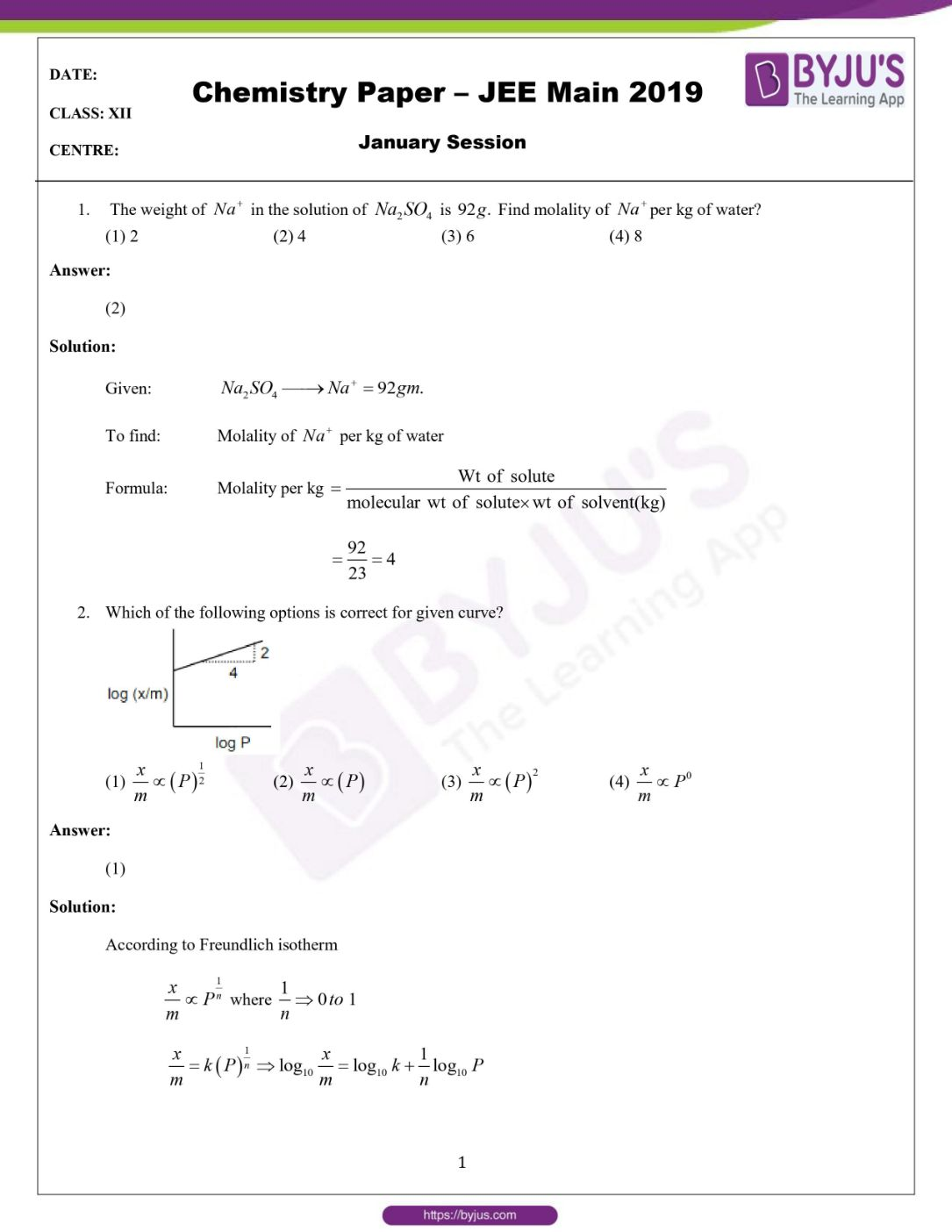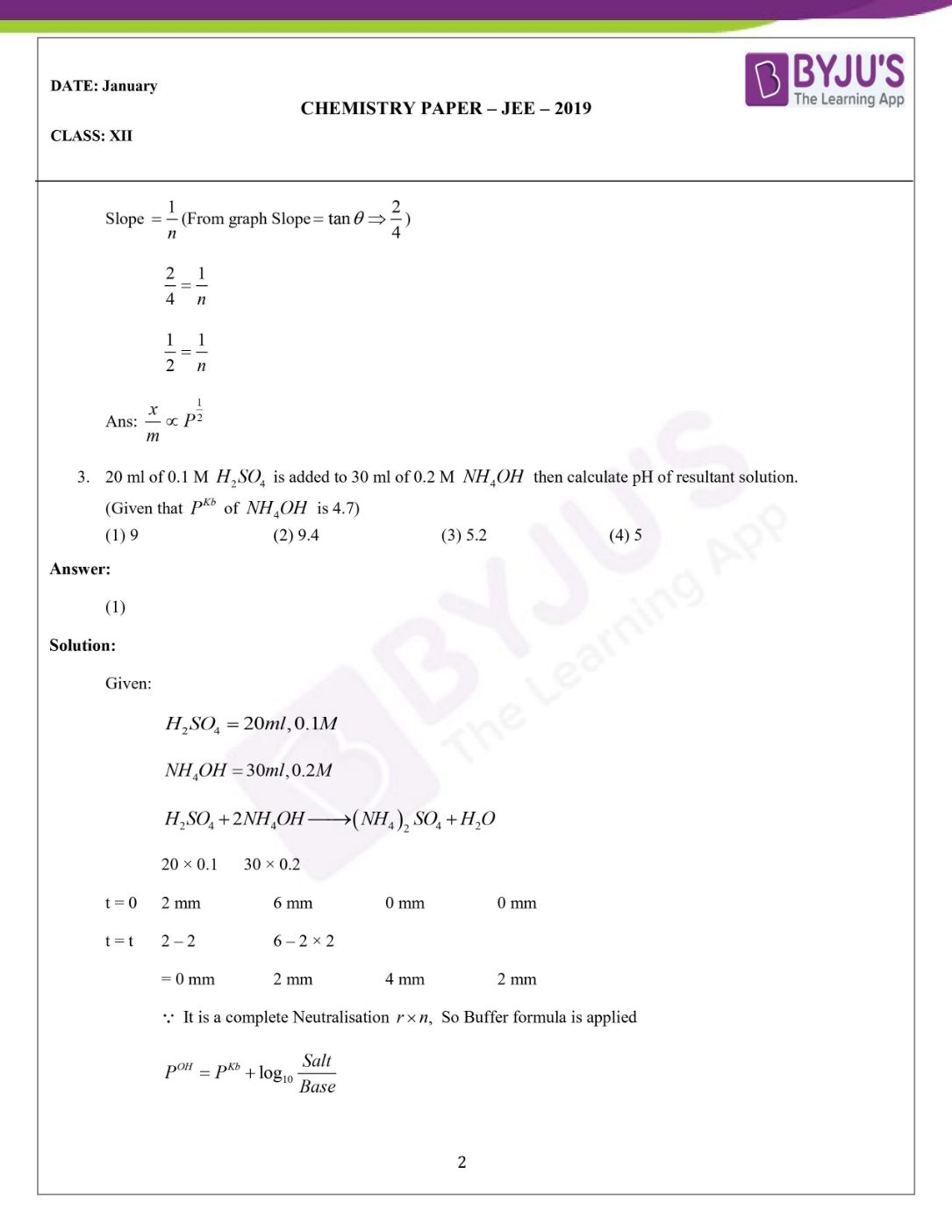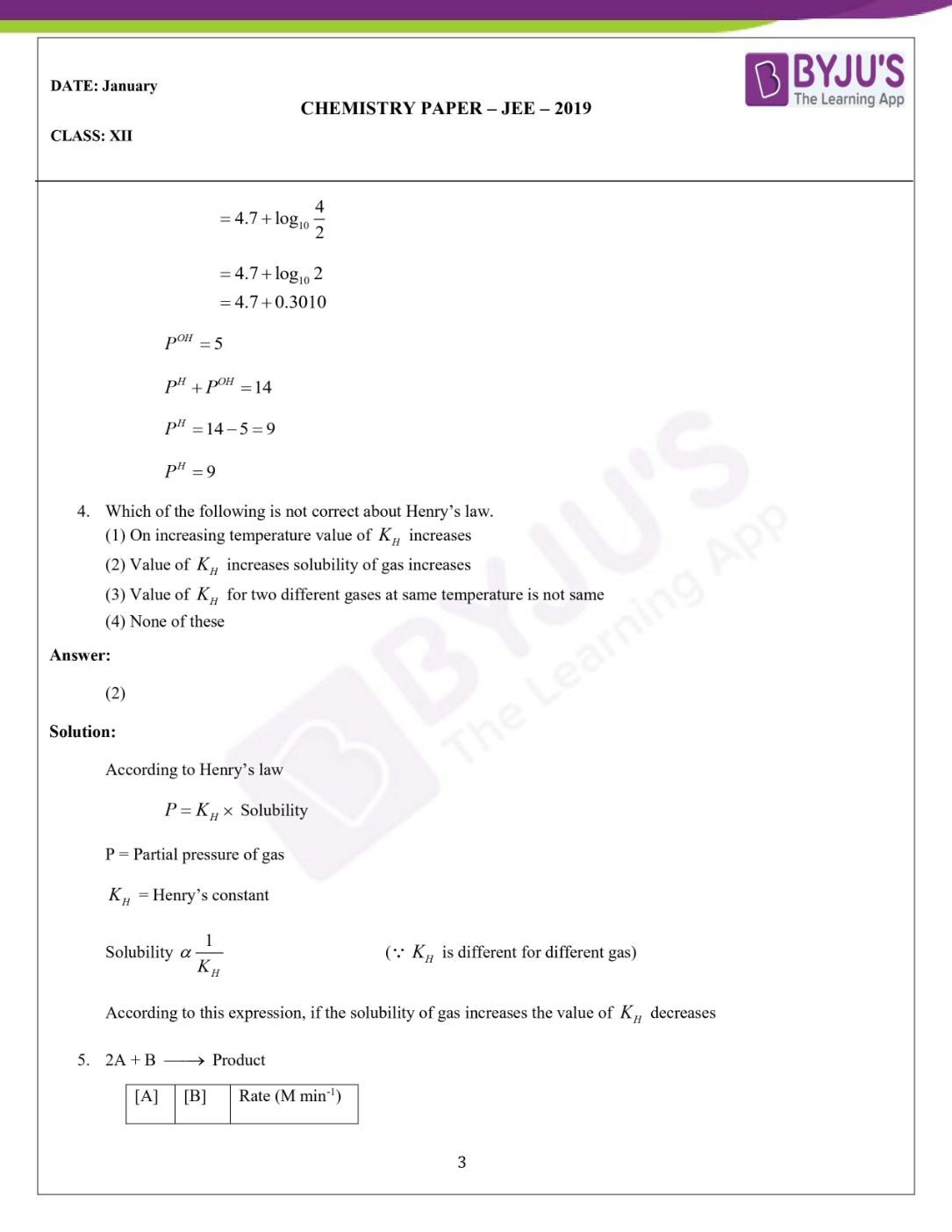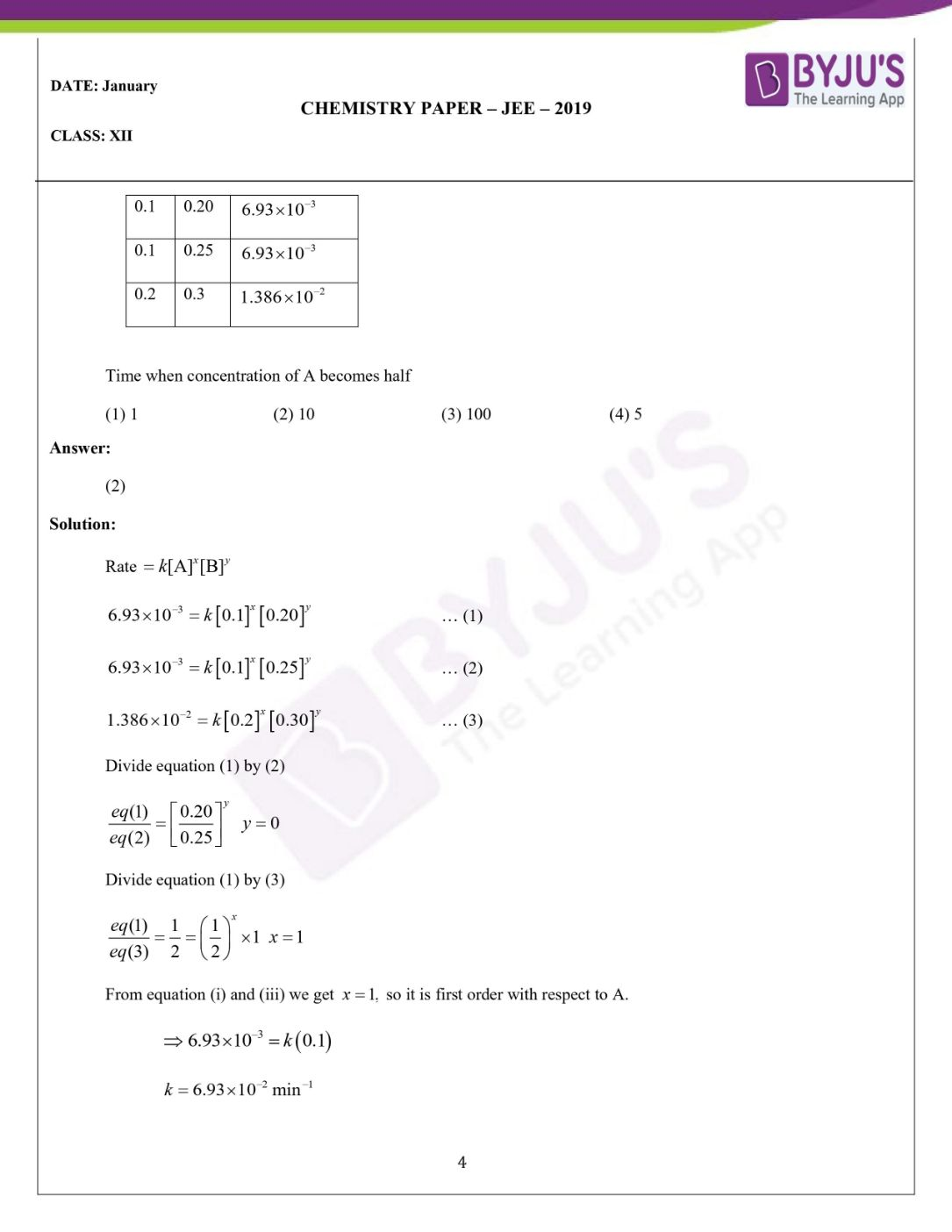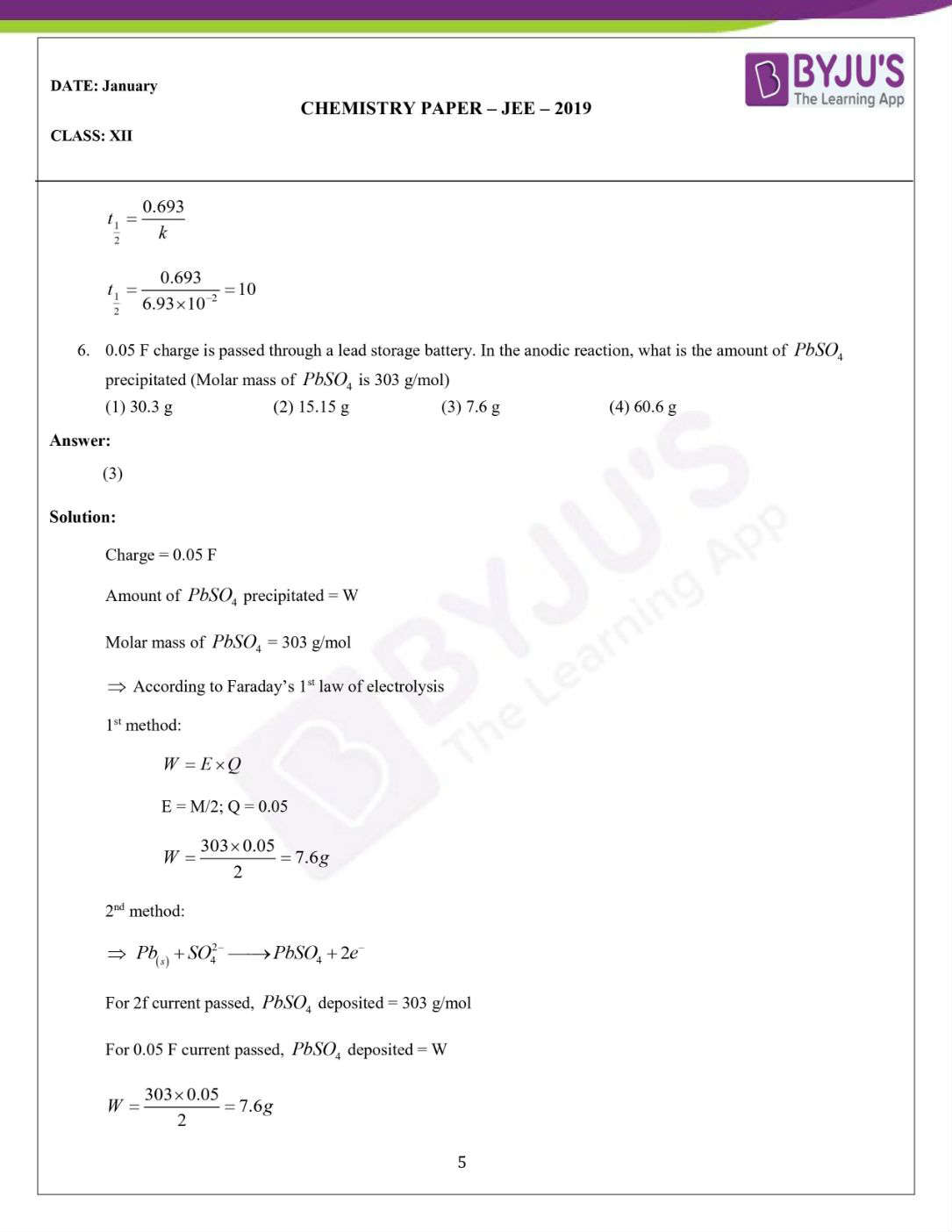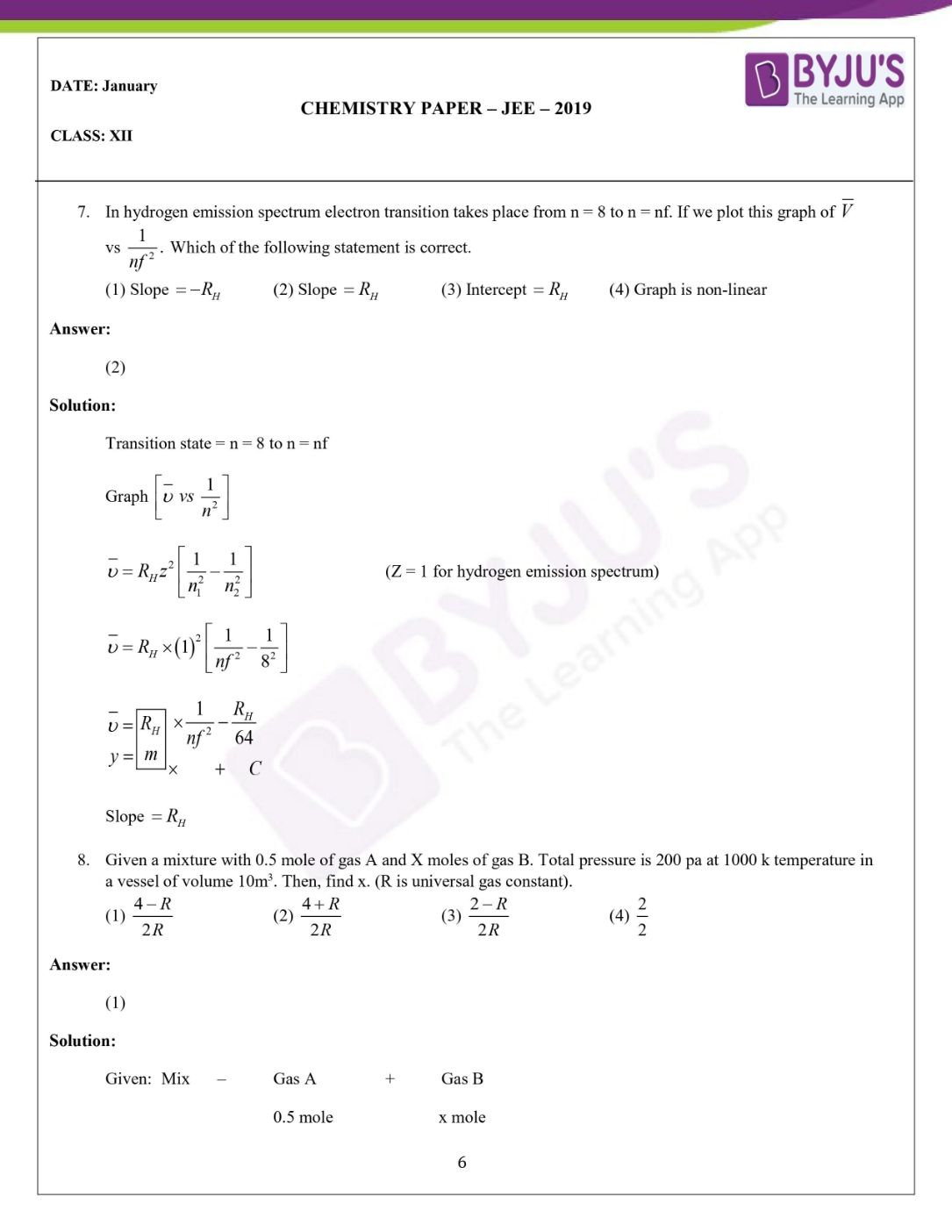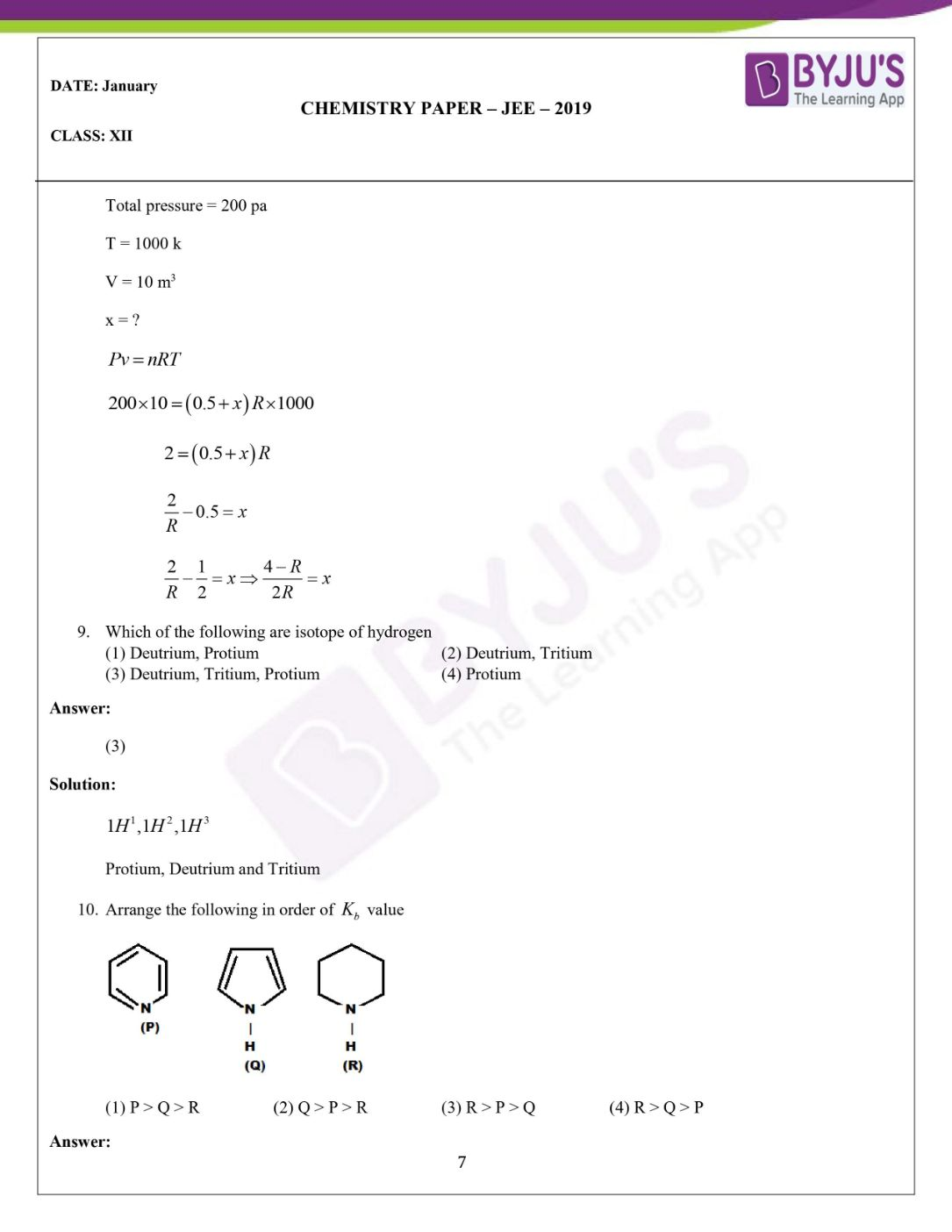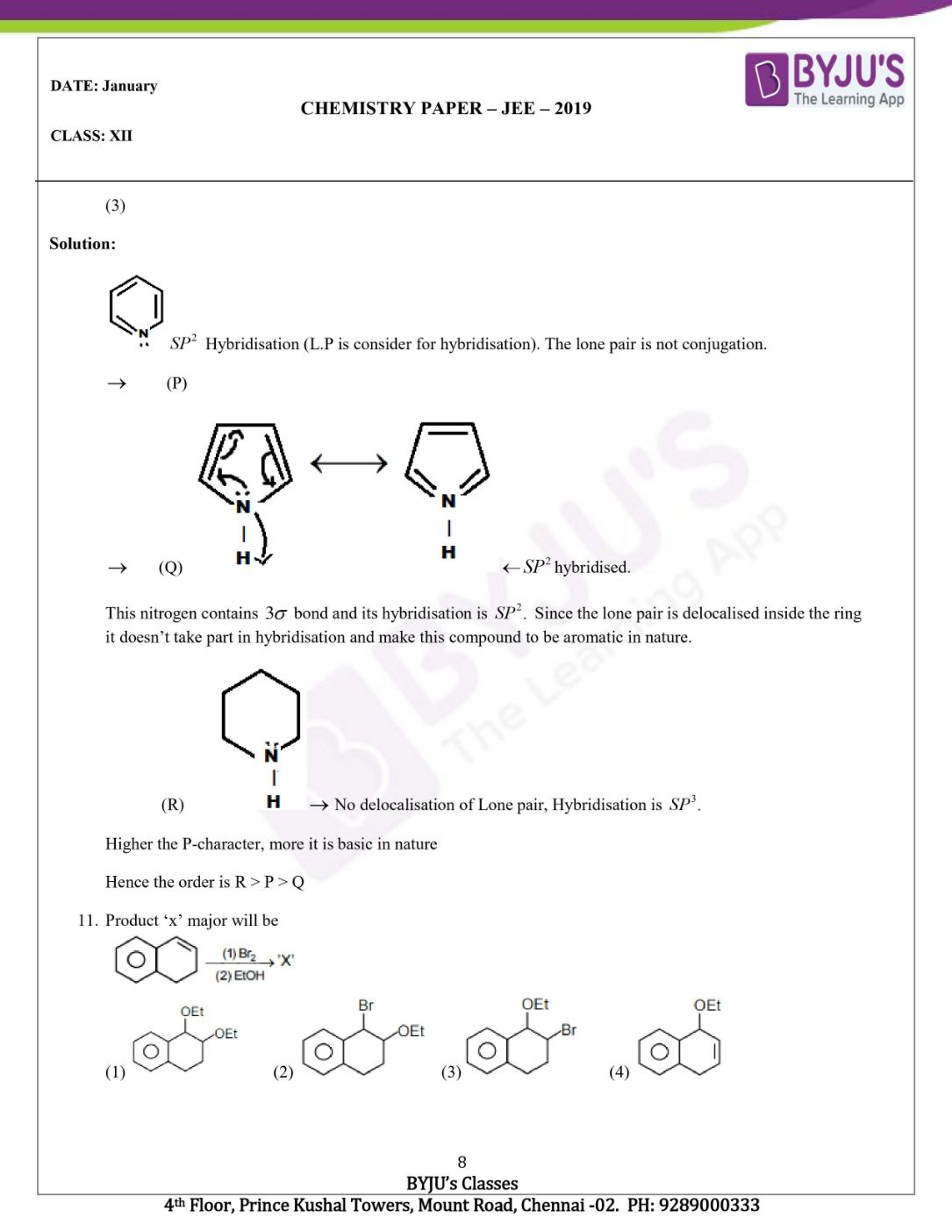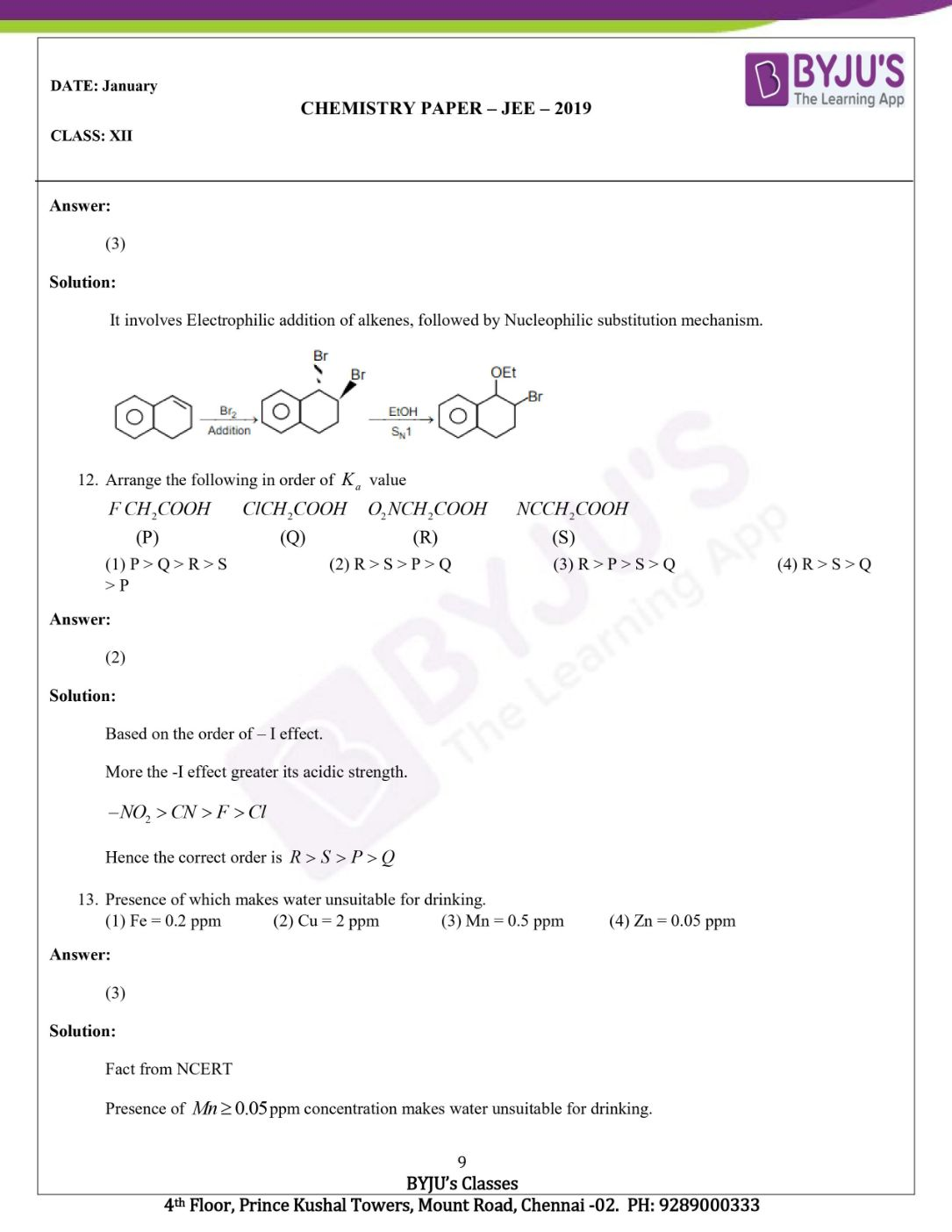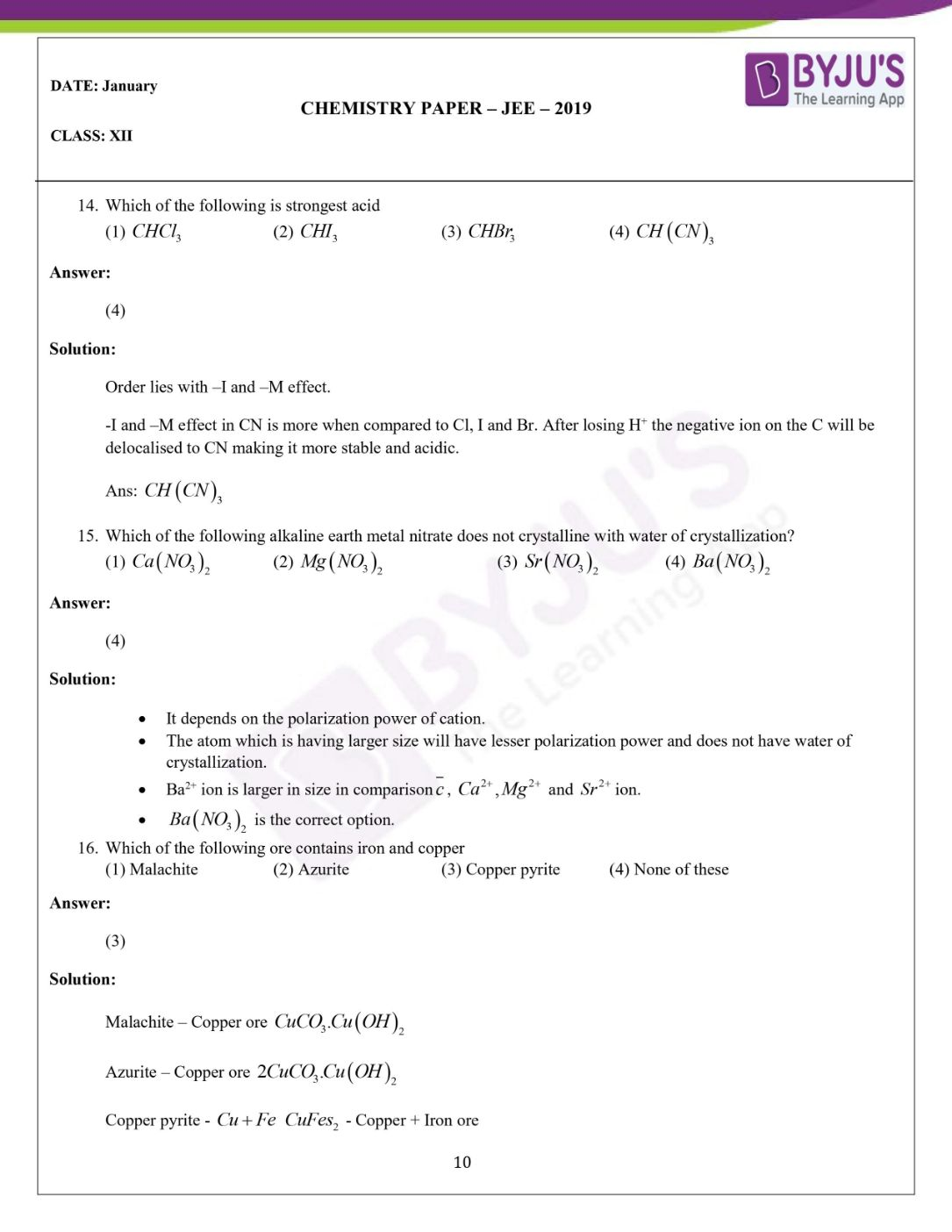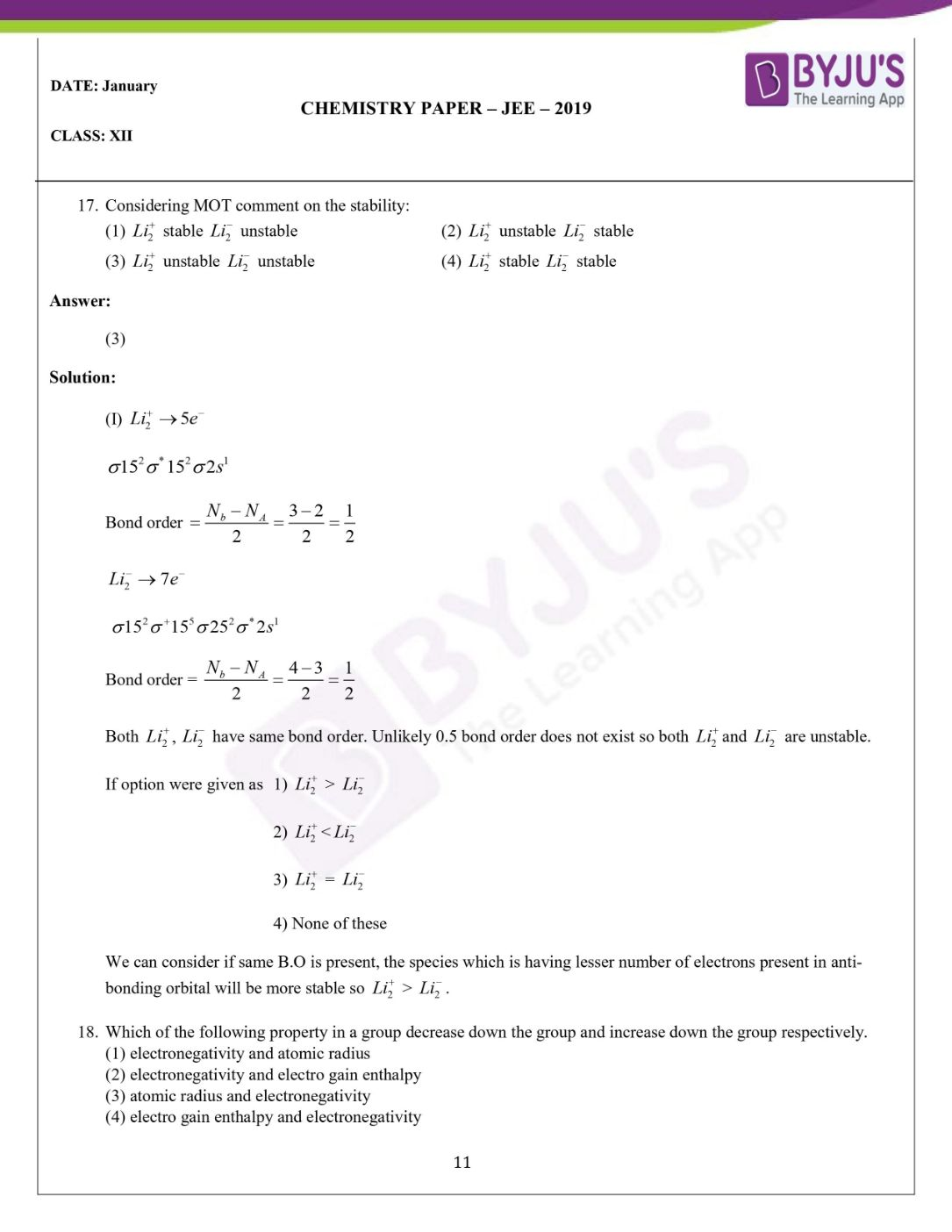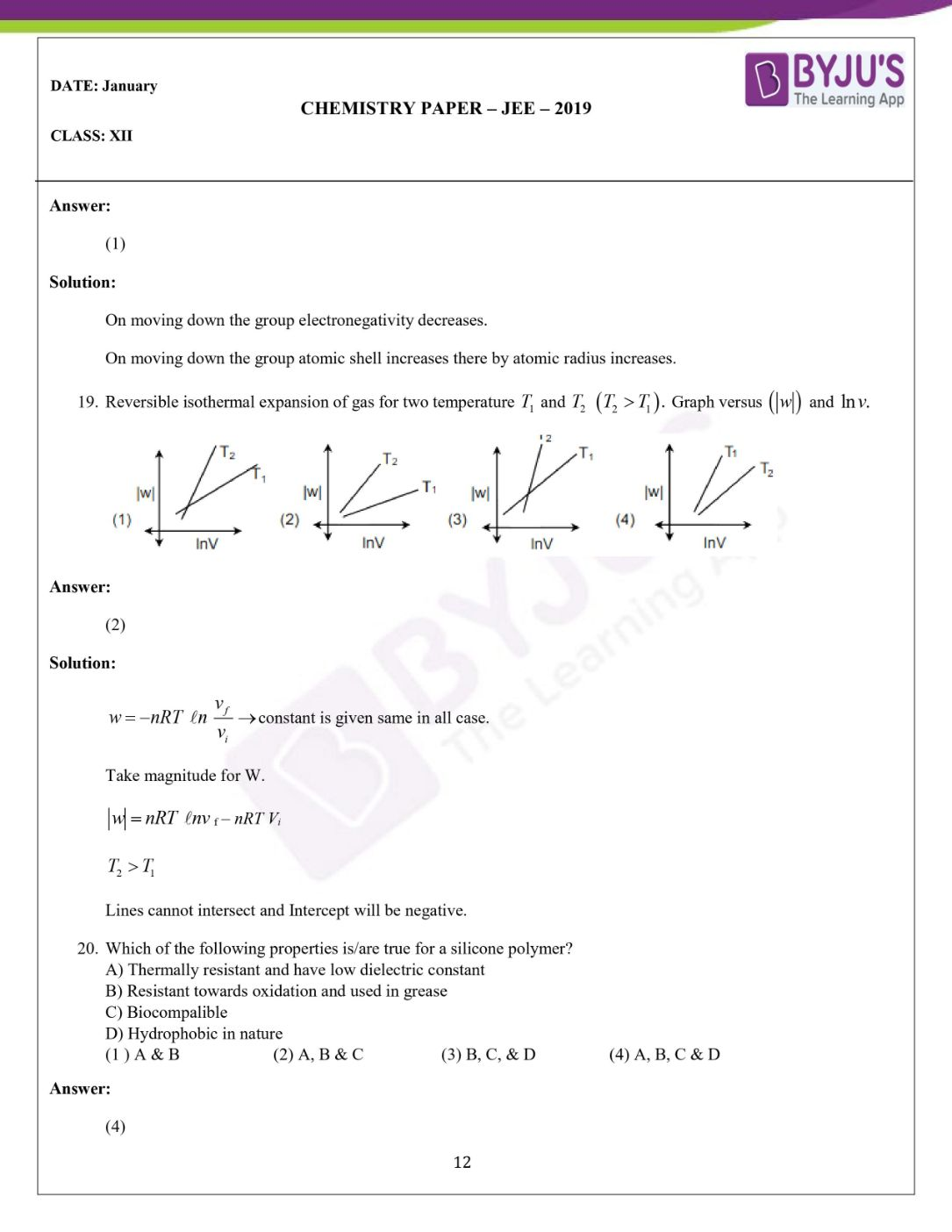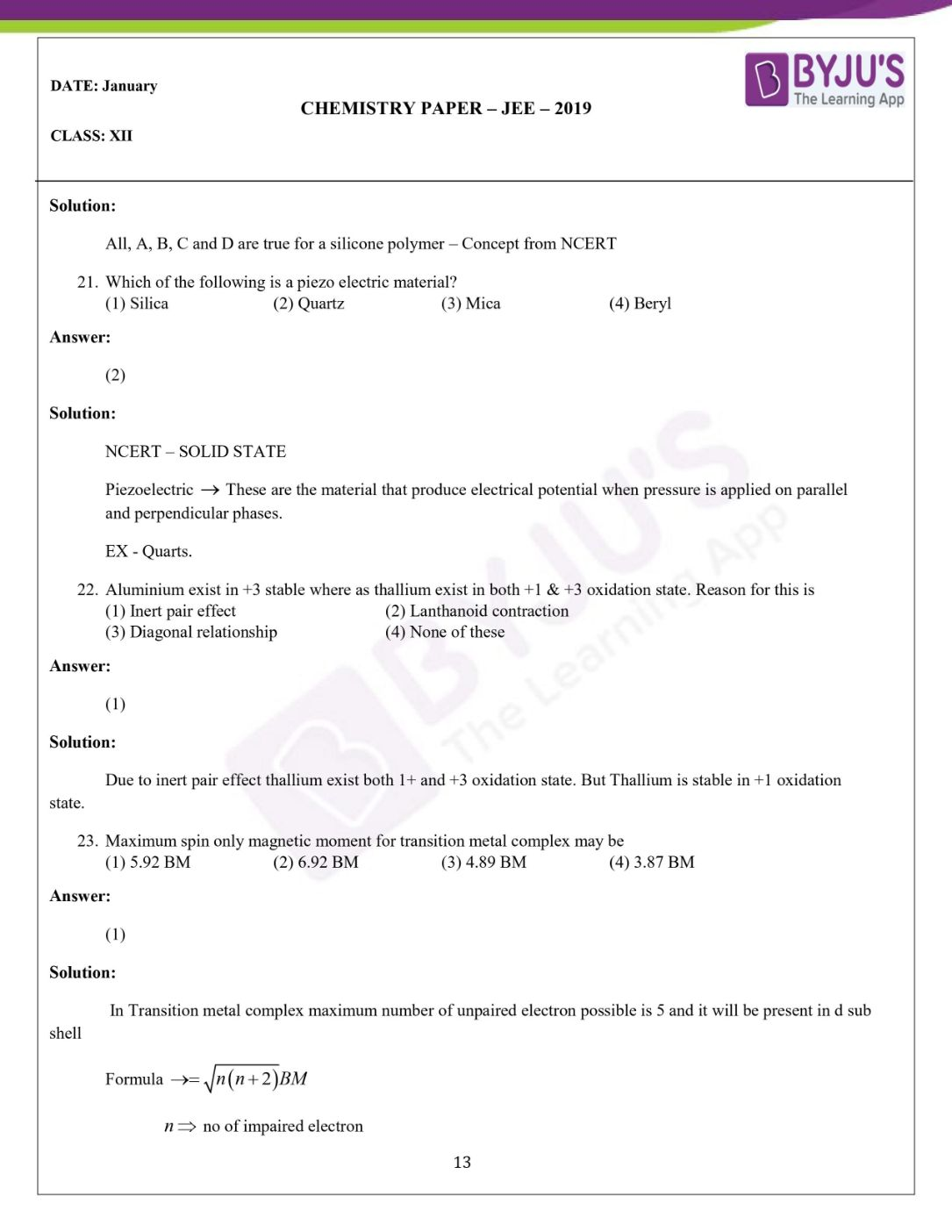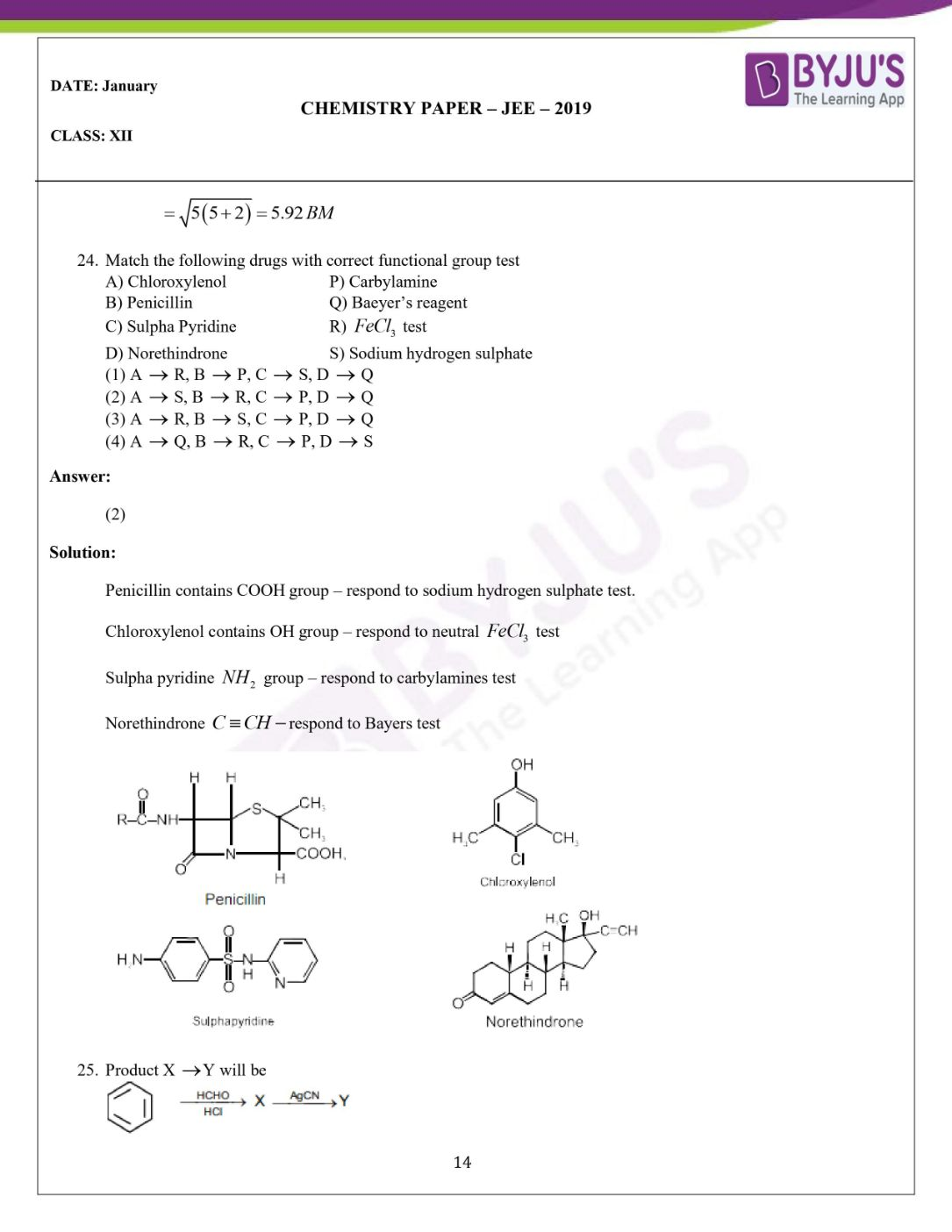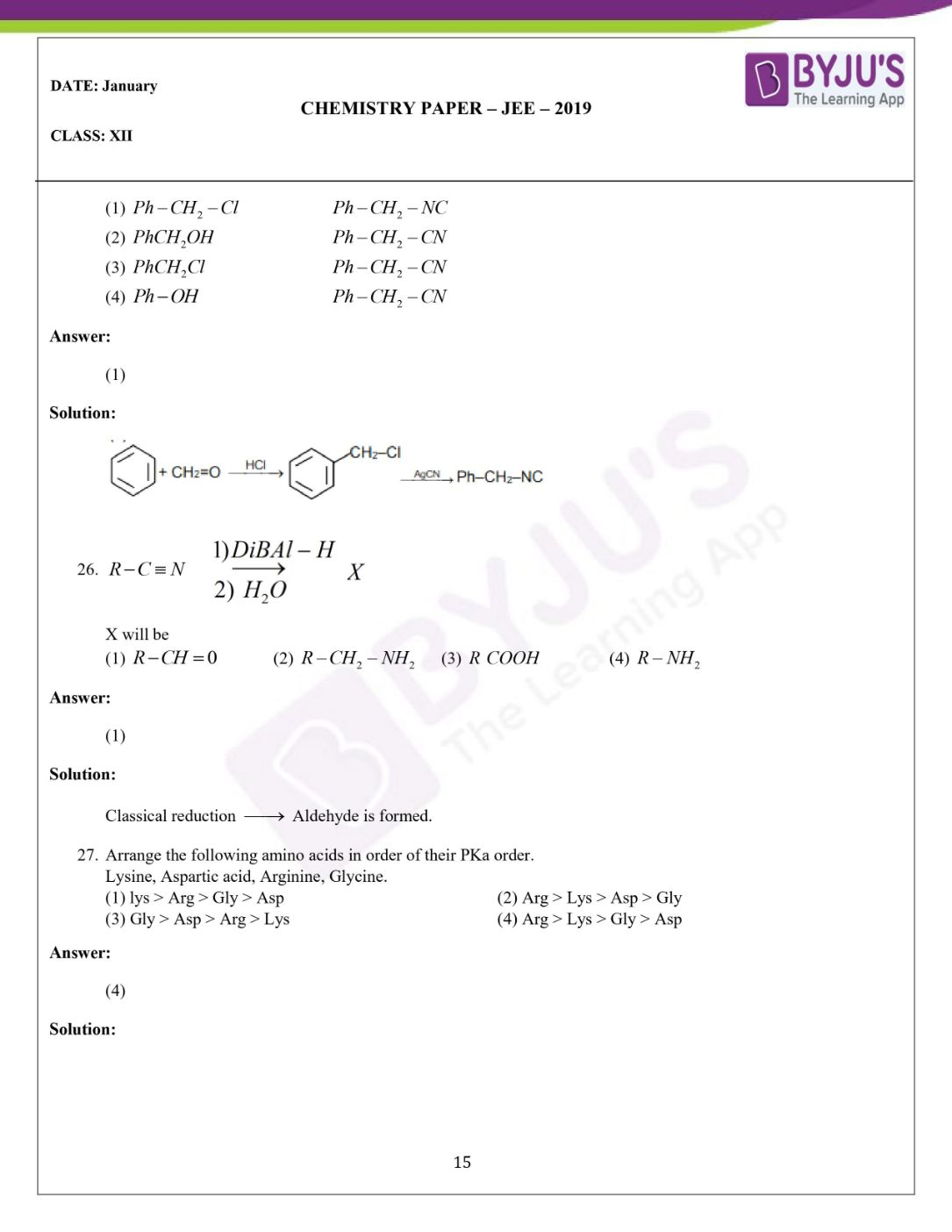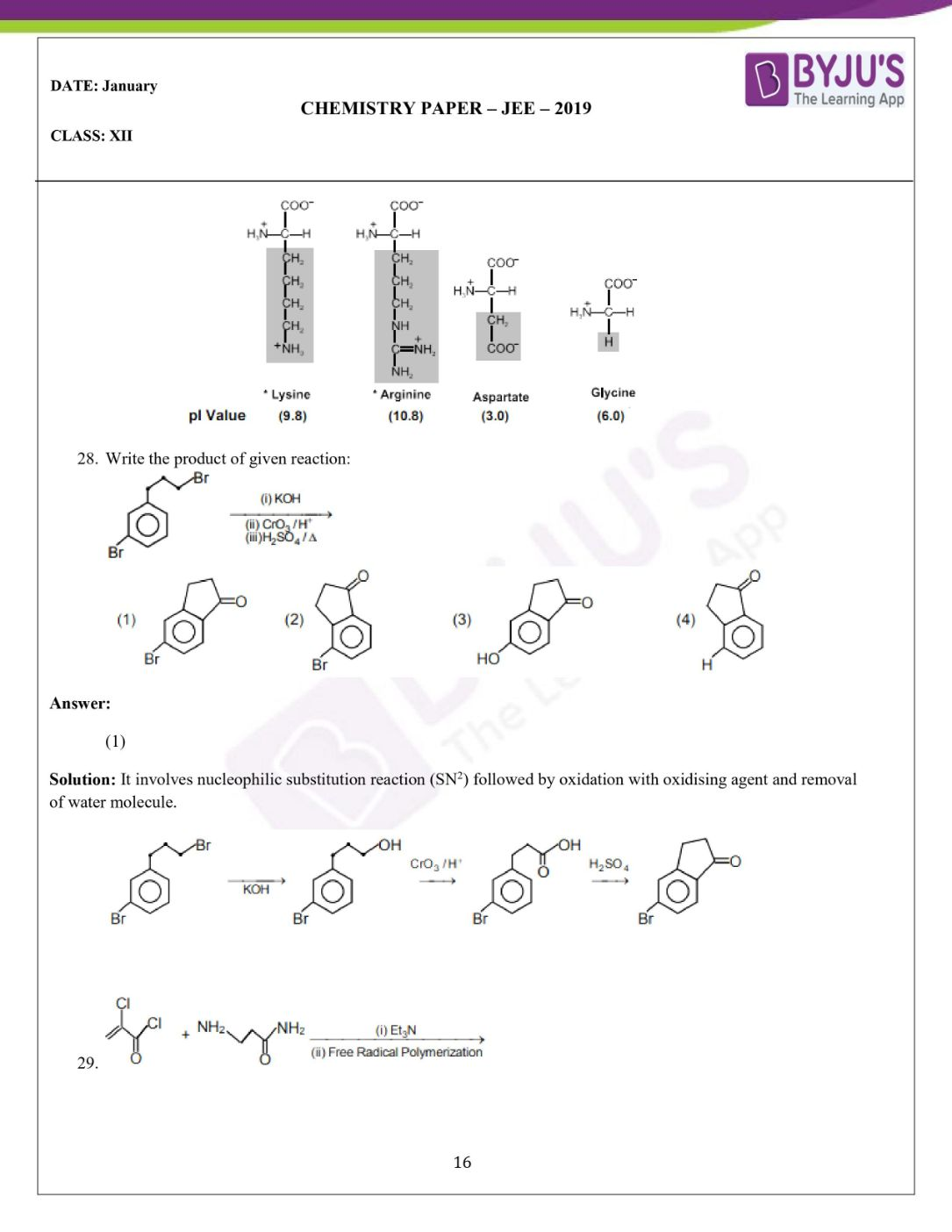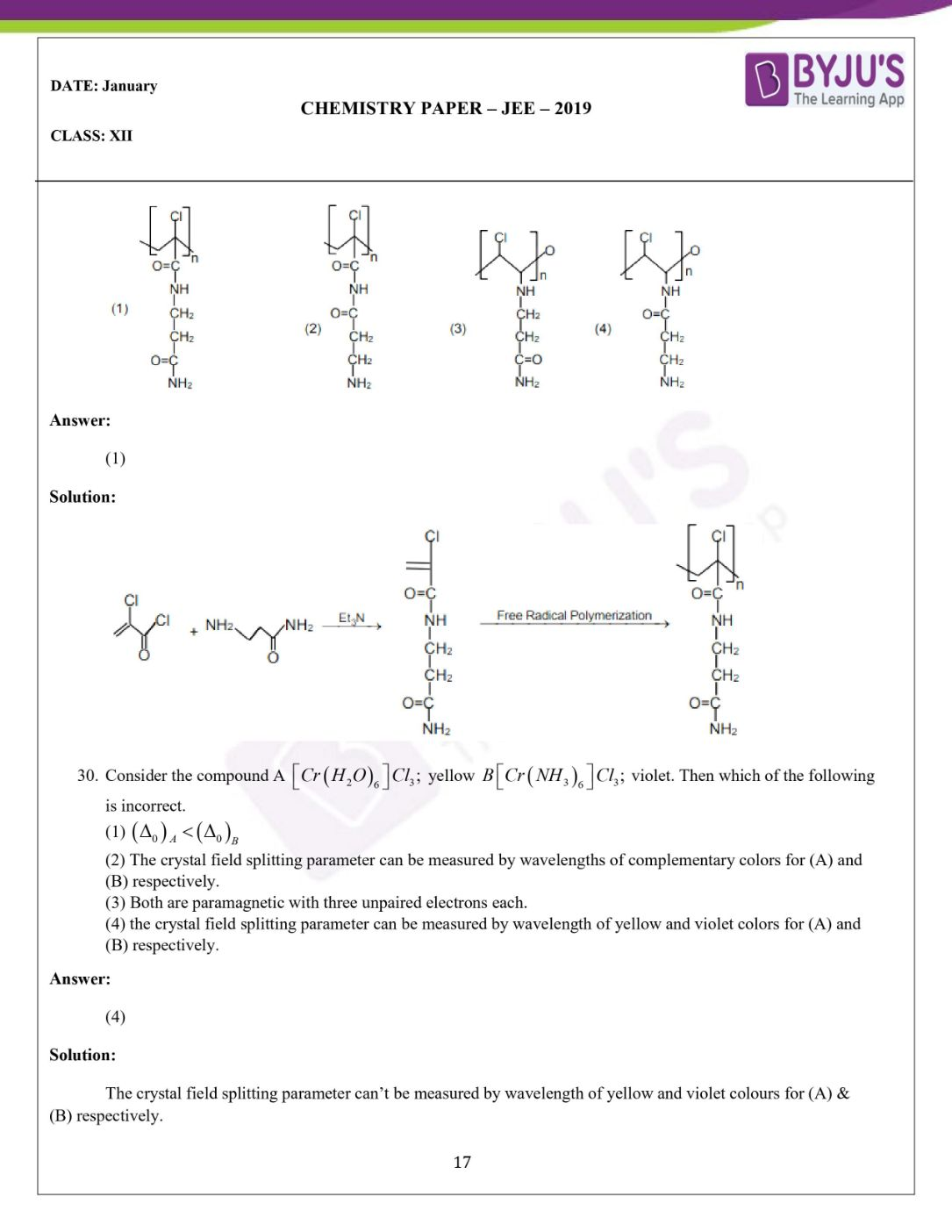Win up to 100% scholarship on Aakash BYJU'S JEE/NEET courses with ABNAT Win up to 100% scholarship on Aakash BYJU'S JEE/NEET courses with ABNAT

# JEE Main 2019 Chemistry Paper With Solutions January Session

JEE Main 2019 Chemistry Paper for January session is solved on this page. The questions have been analyzed by our subject experts and the best and easy to understand solutions have been prepared by them. Studenst can refer to these questions and practise them to understand the pattern of the question paper. Solving these problems will help each JEE aspirant to self analyse their level of preparation and also helps in managing time during the examination. Students can also easily download these question paper and solutions from our website in PDF format.
January 2019 Chemistry Paper
Question 1. The weight of Na+ in the solution of Na2 SO4 is 92g . Find molality of Na+ per kg of water?
a) 2
b) 4
c) 6
d) 8

Given: Na2 SO4. ⎯⎯→ Na+ = 92 gm

To find: Molality of Na+ per kg of water

Formula: Molality per kg = (Wt of solute) / (molecular wt of solute wt of solvent(kg)) = 92/23 = 4

Question 2. Which of the following options is correct for given curve?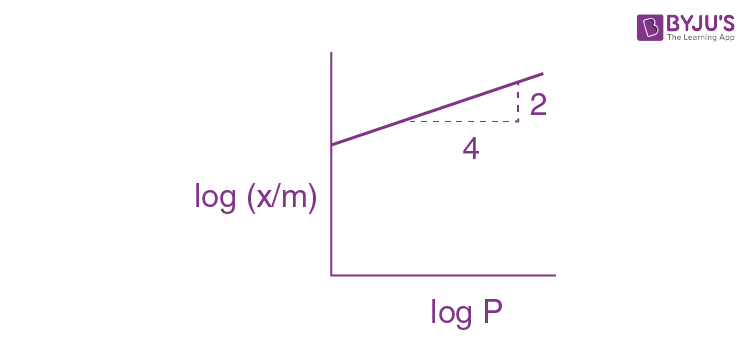a) (x/m) ∝ (P)1/2
b) (x/m) ∝ (P)
c) (x/m) ∝ (P)2
d) (x/m) ∝ (P)0

According to Freundlich isotherm

(x/m) ∝ (P)1/n

Where, 1/n implies 0 to 1

(x/m) = k(P) 1/n

$$\begin{array}{l}log_{10}\frac{x}{m}= log_{10}k+\frac{1}{n}log_{10}P\end{array}$$

Slope= 1/n = (From graph Slope= tan 2/4)

2/4 n = 1/ n

1/2 =1/n

Question 3. 20 ml of 0.1 M H2SO4 is added to 30 ml of 0.2 M NH 4OH then calculate pH of resultant solution.

(Given that P Kb of NH4OH is 4.7)

a) 9
b) 9.4
c) 5.2
d) 5

H2SO4+2 NH4OH → (NH4)2SO4+ H2O

20 × 0.1 ; 30 × 0.2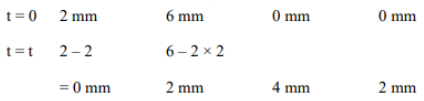It is a complete Neutralisation ,r x n So Buffer formula is applied

POH = Pkb + log10(Salt/Base)

= 4.7 + log10(4/2)

= 4.7 + log10(2)

P OH = 5

PH+POH =14

PH = 14 -5 = 9

PH = 9

Question 4. Which of the following is not correct about Henry’s law.
a) On increasing temperature value of KH increases
b) Value of KH increases solubility of gas increases
c) Value of KH for two different gases at same temperature is not same
d) None of these

According to Henry’s law

P = KH x Solubility

P = Partial pressure of gas

KH = Henry’s constant

Solubility ∝ 1/KH (KH is different for different gas)

According to this expression, if the solubility of gas increases the value of KH decreases.

Question 5. 2A + B → Product

 [A] [B] Rate (M min-1 ) 0.1 0.20 6.93 x 10-3 0.1 0.25 6.93 x 10-3 0.2 0.3 1.386 x 10-2

Time when concentration of A becomes half

a) 1
b) 10
c) 100
d) 5

Rate = k[A] x [B] y

6.93 x 10-3 = k(0.1)x (0.20)y… (1)

6.93 x 10-3 = k(0.1)x (0.25)y… (2)

1.386 x 10-2 = k(0.2)x (0.30)y… (3)

Divide equation (1) by (2)

1= (0.20/0.25)y y = 0

Divide equation (1) by (3)

(1/2) = (1/2)x x 1

x=1

From equation (i) and (iii) we get x =1, so it is first order with respect to A.

6.93 x 10-3 = k(0.1 )

k = 6.93 x 10-2 min-1

t 1/2 = 0.693/k

t 1/2 = 0.693/(6.93 x 10-2) =10

Question 6.0.05 F charge is passed through a lead storage battery. In the anodic reaction, what is the amount of PbSO4 precipitated (Molar mass of PbSO4 is 303 g/mol)
a) 30.3 g
b) 15.5 g
c) 7.6 g
d) 60.6 g

Charge = 0.05 F

Amount of PbSO4 precipitated = W

Molar mass of PbSO4 = 303 g/mol

According to Faraday’s 1st law of electrolysis

1 st method

W = E x Q

E = M/2; Q= 0.05

W = (303 x 0.05)/2 = 7.6 g

2 nd method:

Pb(s) + SO4 2- ⎯⎯→ PbSO4 + 2e

For 2f current passed, PbSO4 deposited = 303 g/mol

For 0.05 F current passed, PbSO4 deposited = W

W = (303x 0.05)/2= 7.6 g

Question 7. In hydrogen emission spectrum electron transition takes place from n = 8 to n = nf. If we plot this graph of ῡ vs (1/nf2 ). Which of the following statement is correct.
a) Slope = −RH
b) Slope = RH
c) Intercept = RH
d) Graph is non-linear

Transition state = n = 8 to n = nf

Graph (ῡ vs (1/nf2 ))

ῡ = RH z2[(1/n12) – (1/n22)] (Z = 1 for hydrogen emission spectrum)

ῡ = RH 12[(1/nf2) – (1/82)]

Comparing it with y = mc2

Slope = RH

Question 8. Given a mixture with 0.5 mole of gas A and X moles of gas B. Total pressure is 200 pa at 1000 k temperature in a vessel of volume 10m3. Then, find x. (R is universal gas constant).
a) (4-R)/2R
b) (4+R)/2R
c) (2-R)/2R
d) 2/2

Given: Mix – Gas A + Gas B

Gas A = 0.5 mole and Gas B = x mole

Total pressure = 200 pa

T = 1000 k V = 10 m3

x = ?

Pv = nRT

200 x 10 = (0.5+ x )R x 1000

2 = (0.5 + x) R

(2/R) -0.5 = x

(2/R) – (1/2) = x (4-R/2R) = x

Question 9.Which of the following are isotope of hydrogen
a) Deutrium, Protium
b) Deutrium, Tritium
c) Deutrium, Tritium, Protium
d) Protium

1H1,1H2,1H3

Protium, Deutrium and Tritium

Question 10. Arrange the following in order of Kb valuea) P > Q > R
b) Q > P > R
c) R > P > Q
d) R > Q > P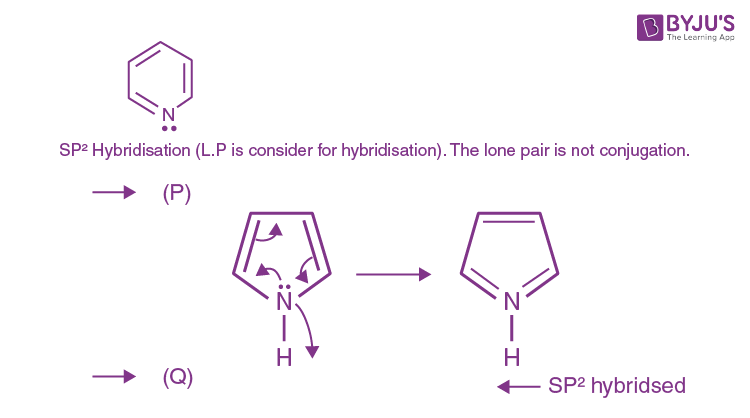This nitrogen contains 3σ bond and its hybridisation is SP2 . Since the lone pair is delocalised inside the ring it doesn’t take part in hybridisation and make this compound to be aromatic in nature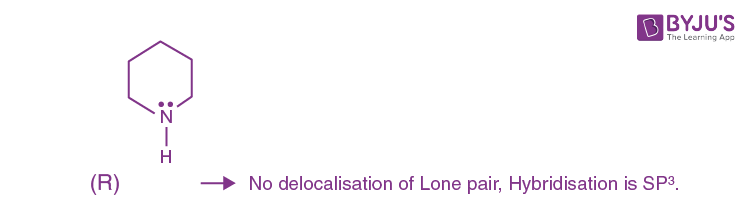Question 11.Product ‘x’ major will be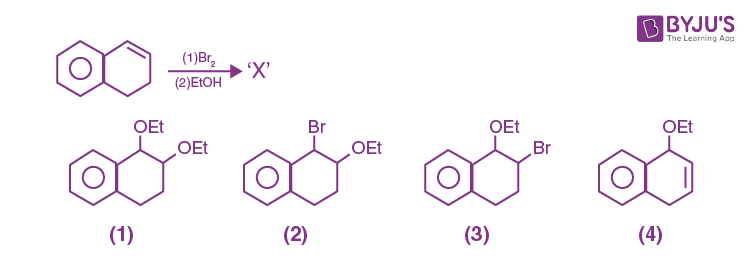It involves Electrophilic addition of alkenes, followed by Nucleophilic substitution mechanism.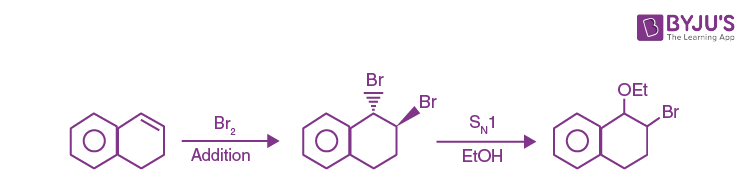Question 12. Arrange the following in order of K a value

(P) FCH2COOH

(Q) ClCH2COOH

(R) O2NCH2COOH

(S) NCCH2COOH

a) P > Q > R > S
b) R > S > P > Q
c) R > P > S > Q
d) R > S > Q > P

Based on the order of – I effect.

More the -I effect greater its acidic strength.

-NO2 >CN> F >Cl

Hence the correct order is R > S > P > Q

Question 13.Presence of which makes water unsuitable for drinking.
a) Fe = 0.2 ppm
b) Cu = 2 ppm
c) Mn = 0.5 ppm
d) Zn = 0.05 ppm

Fact from NCERT

Presence of Mn ≥ 0.05 ppm concentration makes water unsuitable for drinking

Question 14. Which of the following is strongest acid
a) CHCl3
b) CHI3
c) CHBr3
d) CH (CN)3

Order lies with –I and –M effect. -I and –M effect in CN is more when compared to Cl, I and Br. After losing H+ the negative ion on the C will be delocalized to CN making it more stable and acidic.

Ans: CH (CN)3

Question 15. Which of the following alkaline earth metal nitrate does not crystalline with water of crystallization?
a) Ca(NO3)2
b) Mg(NO3)2
c) Sr(NO3)2
d) Ba(NO3)2

•It depends on the polarization power of cation.

• The atom which is having larger size will have lesser polarization power and does not have water of crystallization.

• Ba 2+ ion is larger in size in comparison to Ca 2+ Mg 2+ and Sr 2+ ion.

• Ba(NO3)2 is the correct option.

Question 16. Which of the following ore contains iron and copper

a) Malachite
b) Azurite
c) Copper pyrite
d) None of these

Malachite – Copper ore CuCO3 Cu(OH)2 .

Azurite – Copper ore 2CuCO3 Cu(OH)2

Copper pyrite – Cu+ Fe CuFes2 – Copper + Iron ore

Question 17. Considering MOT comment on the stability:

a) Li2+ stable Li2unstable
b) Li2+ unstable Li2unstable
c) Li2+ unstable Li2 unstable
d) Li2+ stable Li2 stable

Li2+ → 5e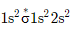Bond order = (Nb –NA)/2 = (3-2)/2 =1/2

Li2 → 7e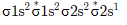Bond order = (Nb –NA)/2 = (4-3)/2 =1/2

Both Li2+, + Li2 have same bond order. Unlikely 0.5 bond order does not exist so both Li2+ and Li2 are unstable.

If option were given as

1) Li2+ > Li2

2) Li2+ < Li2

3) Li2+ = Li2

4) None of these

We can consider if same B.O is present, the species which is having lesser number of electrons present in antibonding orbital will be more stable so Li2+ > Li2 .

Question 18. Which of the following property in a group decrease down the group and increase down the group respectively.
b) electronegativity and electro gain enthalpy
d) electro gain enthalpy and electronegativity

On moving down, the group electro negativity decreases. On moving down, the group atomic shell increases there by atomic radius increases.

Question 19. Reversible isothermal expansion of gas for two temperature T1 and T2 (T2 > T1) Graph versus (
$$\begin{array}{l}\left | w \right |\end{array}$$
) and ln v.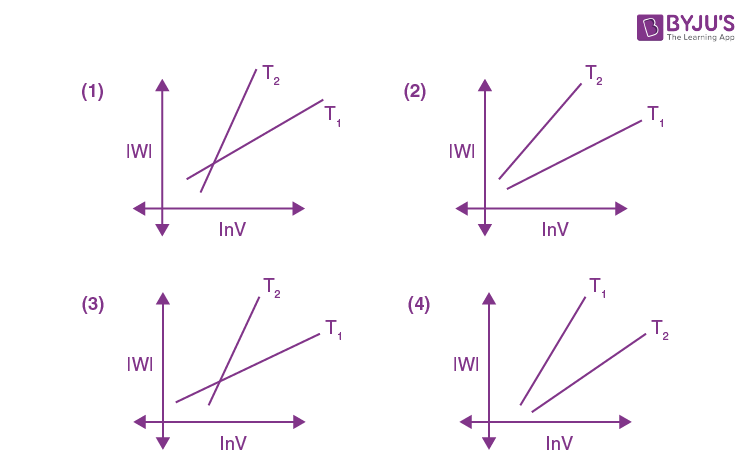w = – nRT ln (vf/vi) → constant is given same in all case.

Take magnitude forW.

(

$$\begin{array}{l}\left | w \right |\end{array}$$
) = nRT lnvf – nRT Vi

T2 > T1

Lines cannot intersect and Intercept will be negative.

Question 20. Which of the following properties is/are true for a silicone polymer?

A) Thermally resistant and have low dielectric constant

B) Resistant towards oxidation and used in grease

C) Biocompatible

D) Hydrophobic in nature

a) A & B
b) A, B & C
c) B, C, & D
d) A, B, C & D

All, A, B, C and D are true for a silicone polymer

Question 21. Which of the following is a piezo electric material?

a) Silica)
b) Quartz
c) Mica
d) Beryl

Piezoelectric → these are the material that produce electrical potential when pressure is applied on parallel and perpendicular phases.

EX – Quarts.

Question 22.Aluminium exist in +3 stable whereas thallium exists in both +1 & +3 oxidation state. Reason for this is;
a) Inert pair effect
b) Lanthanoid contraction
c) Diagonal relationship
d) None of these

Due to inert pair effect thallium exist both 1+ and +3 oxidation state. But Thallium is stable in +1 oxidation state.

Question 23. Maximum spin only magnetic moment for transition metal complex may be
a) 5.92 BM
b) 6.92 BM
c) 4.89 BM
d) 3.87 BM

In Transition metal complex maximum number of unpaired electron possible is 5 and it will be present in d sub shell

Formula → =

$$\begin{array}{l}\sqrt{n(n+2)}BM\end{array}$$

n no of impaired electron

=

$$\begin{array}{l}\sqrt{5(5+2)}BM\end{array}$$
= 5.92 BM

Question 24. Match the following drugs with correct functional group test

A) Chloroxylenol P) Carbylamines

B) Penicillin Q) Baeyer’s reagent

C) Sulpha Pyridine R) FeCl3 test

D) Norethindrone S) Sodium hydrogen sulphate

a) A → R, B → P, C → S, D → Q
b) A → S, B → R, C → P, D → Q
c) A → R, B → S, C → P, D → Q
d) A → Q, B → R, C → P, D → S

Penicillin contains COOH group – respond to sodium hydrogen sulphate test.

Chloroxylenol contains OH group – respond to neutral FeCl3 test

Sulpha pyridine NH2 group – respond to carbylamines test

Norethindrone C ≡ CH – respond to Bayers test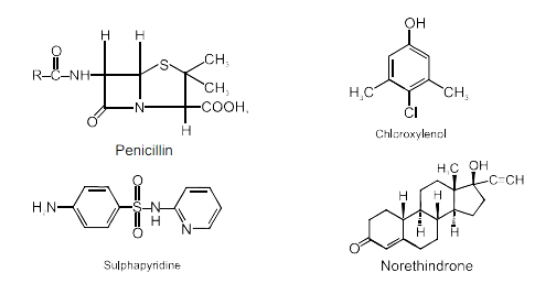Question 25.Product X → Y will bea) Ph − CH − Cl       Ph− CH2− NC
b) PhCH2OH       Ph− CH2 − CN
c) PhCH2 Cl       Ph− CH2− CN
d) Ph − OH       Ph− CH2− CN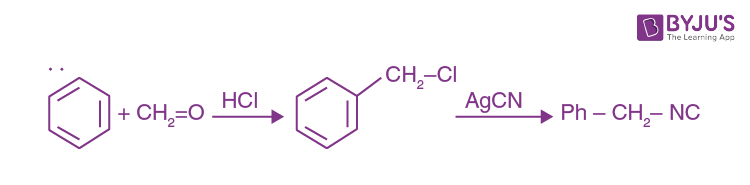Question In the given image, X will be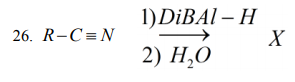a) R-CH=0
b) R-CH2NH2
c) R COOH
d) R-NH2

Classical reduction ⎯⎯→ Aldehyde is formed.

Question 27.Arrange the following amino acids in order of their PKa order.

Lysine, Aspartic acid, Arginine, Glycine.

a) lys > Arg > Gly > Asp
b) Arg > Lys > Asp > Gly
c) Gly > Asp > Arg > Lys
d) Arg > Lys > Gly > Asp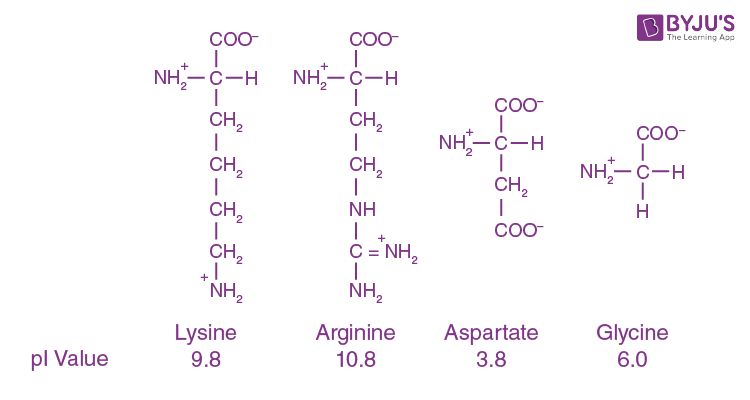Question 28.Write the product of given reaction: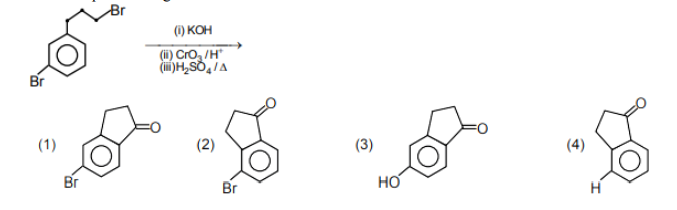It involves nucleophilic substitution reaction (SN2 ) followed by oxidation with oxidising agent and removal of water molecule.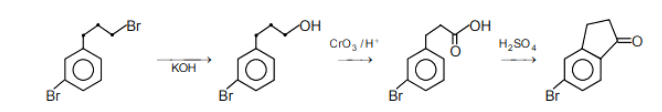Question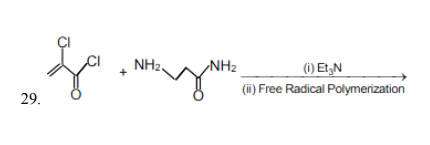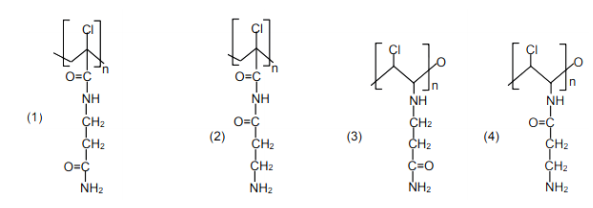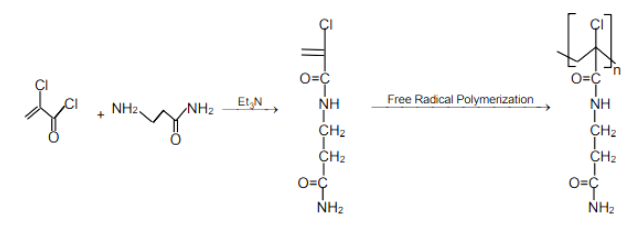Question 30.Consider the compound A [Cr (H2O)6] Cl3 ; yellow B [Cr(NH3)6] Cl3 violet. Then which of the following is incorrect.
a) (Δ0)A< (Δ0)B
b) The crystal field splitting parameter can be measured by wavelengths of complementary colors for (A) and (B) respectively.
c) Both are paramagnetic with three unpaired electrons each.
d) The crystal field splitting parameter can be measured by wavelength of yellow and violet colors for (A) and (B) respectively.

The crystal field splitting parameter can’t be measured by wavelength of yellow and violet colours for (A) & (B) respectively.